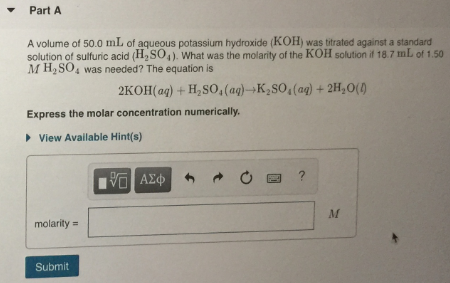# A volume of 50.0 mL of aqueous potassium hydroxide (KOH) was titrated against a standard solution of sulfuric acid (H2SO4). What was the molarity of the KOH solution if 18.7 mL of 1.50 M H2SO4 was needed? The equation is 2KOH(aq) + H2SO4(aq) → K2SO4(aq) + 2H2O(I). Express the molar concentration numerically.